QuTiP example: Quantum Gates and their usage¶

Author: Anubhav Vardhan ([email protected])

User-defined gate added by: Boxi Li ([email protected])

Installation:¶

The circuit image visualization requires LaTeX and ImageMagick for display. The module automatically process the LaTeX code for plotting the circuit, generate the pdf and convert it to the png format. On Mac and Linux, ImageMagick can be easily installed with the command conda install imagemagick if you have conda installed. Otherwise, please follow the installation instructions on the ImageMagick documentation.

On windows, you need to download and install ImageMagick installer. In addition, you also need perl (for pdfcrop) and Ghostscript (additional dependency of ImageMagick for png conversion).

To test if the installation is complete, try the following three commands working correctly in Command Prompt: pdflatex, pdfcrop and magick anypdf.pdf antpdf.png, where anypdf.pdf is any pdf file you have.

In :
%matplotlib inline
In :
from IPython.display import Image
In :
from numpy import pi
import numpy as np
In :
from qutip import *
from qutip_qip.operations import *
from qutip_qip.circuit import QubitCircuit, Gate

Gates in QuTiP and their representation¶

Controlled-PHASE¶

In :
cphase(pi/2)
Out:
Quantum object: dims = [[2, 2], [2, 2]], shape = (4, 4), type = oper, isherm = False\begin{equation*}\left(\begin{array}{*{11}c}1.0 & 0.0 & 0.0 & 0.0\\0.0 & 1.0 & 0.0 & 0.0\\0.0 & 0.0 & 1.0 & 0.0\\0.0 & 0.0 & 0.0 & 1.0j\\\end{array}\right)\end{equation*}
In :
q = QubitCircuit(2, reverse_states=False)
q.png
Out: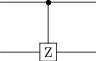In :
rx(pi/2)
Out:
Quantum object: dims = [, ], shape = (2, 2), type = oper, isherm = False\begin{equation*}\left(\begin{array}{*{11}c}0.707 & -0.707j\\-0.707j & 0.707\\\end{array}\right)\end{equation*}
In :
q = QubitCircuit(1, reverse_states=False)
q.png
Out: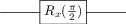In :
ry(pi/2)
Out:
Quantum object: dims = [, ], shape = (2, 2), type = oper, isherm = False\begin{equation*}\left(\begin{array}{*{11}c}0.707 & -0.707\\0.707 & 0.707\\\end{array}\right)\end{equation*}
In :
q = QubitCircuit(1, reverse_states=False)
q.png
Out: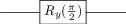In :
rz(pi/2)
Out:
Quantum object: dims = [, ], shape = (2, 2), type = oper, isherm = False\begin{equation*}\left(\begin{array}{*{11}c}(0.707-0.707j) & 0.0\\0.0 & (0.707+0.707j)\\\end{array}\right)\end{equation*}
In :
q = QubitCircuit(1, reverse_states=False)
q.png
Out: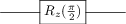CNOT¶

In :
cnot()
Out:
Quantum object: dims = [[2, 2], [2, 2]], shape = (4, 4), type = oper, isherm = True\begin{equation*}\left(\begin{array}{*{11}c}1.0 & 0.0 & 0.0 & 0.0\\0.0 & 1.0 & 0.0 & 0.0\\0.0 & 0.0 & 0.0 & 1.0\\0.0 & 0.0 & 1.0 & 0.0\\\end{array}\right)\end{equation*}
In :
q = QubitCircuit(2, reverse_states=False)
q.png
Out: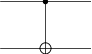CSIGN¶

In :
csign()
Out:
Quantum object: dims = [[2, 2], [2, 2]], shape = (4, 4), type = oper, isherm = True\begin{equation*}\left(\begin{array}{*{11}c}1.0 & 0.0 & 0.0 & 0.0\\0.0 & 1.0 & 0.0 & 0.0\\0.0 & 0.0 & 1.0 & 0.0\\0.0 & 0.0 & 0.0 & -1.0\\\end{array}\right)\end{equation*}
In :
q = QubitCircuit(2, reverse_states=False)
q.png
Out: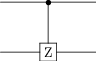Berkeley¶

In :
berkeley()
Out:
Quantum object: dims = [[2, 2], [2, 2]], shape = (4, 4), type = oper, isherm = False\begin{equation*}\left(\begin{array}{*{11}c}0.924 & 0.0 & 0.0 & 0.383j\\0.0 & 0.383 & 0.924j & 0.0\\0.0 & 0.924j & 0.383 & 0.0\\0.383j & 0.0 & 0.0 & 0.924\\\end{array}\right)\end{equation*}
In :
q = QubitCircuit(2, reverse_states=False)
q.png
Out: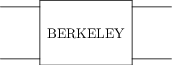SWAPalpha¶

In :
swapalpha(pi/2)
Out:
Quantum object: dims = [[2, 2], [2, 2]], shape = (4, 4), type = oper, isherm = False\begin{equation*}\left(\begin{array}{*{11}c}1.0 & 0.0 & 0.0 & 0.0\\0.0 & (0.610-0.488j) & (0.390+0.488j) & 0.0\\0.0 & (0.390+0.488j) & (0.610-0.488j) & 0.0\\0.0 & 0.0 & 0.0 & 1.0\\\end{array}\right)\end{equation*}

FREDKIN¶

In :
fredkin()
Out:
Quantum object: dims = [[2, 2, 2], [2, 2, 2]], shape = (8, 8), type = oper, isherm = True\begin{equation*}\left(\begin{array}{*{11}c}1.0 & 0.0 & 0.0 & 0.0 & 0.0 & 0.0 & 0.0 & 0.0\\0.0 & 1.0 & 0.0 & 0.0 & 0.0 & 0.0 & 0.0 & 0.0\\0.0 & 0.0 & 1.0 & 0.0 & 0.0 & 0.0 & 0.0 & 0.0\\0.0 & 0.0 & 0.0 & 1.0 & 0.0 & 0.0 & 0.0 & 0.0\\0.0 & 0.0 & 0.0 & 0.0 & 1.0 & 0.0 & 0.0 & 0.0\\0.0 & 0.0 & 0.0 & 0.0 & 0.0 & 0.0 & 1.0 & 0.0\\0.0 & 0.0 & 0.0 & 0.0 & 0.0 & 1.0 & 0.0 & 0.0\\0.0 & 0.0 & 0.0 & 0.0 & 0.0 & 0.0 & 0.0 & 1.0\\\end{array}\right)\end{equation*}

TOFFOLI¶

In :
toffoli()
Out:
Quantum object: dims = [[2, 2, 2], [2, 2, 2]], shape = (8, 8), type = oper, isherm = True\begin{equation*}\left(\begin{array}{*{11}c}1.0 & 0.0 & 0.0 & 0.0 & 0.0 & 0.0 & 0.0 & 0.0\\0.0 & 1.0 & 0.0 & 0.0 & 0.0 & 0.0 & 0.0 & 0.0\\0.0 & 0.0 & 1.0 & 0.0 & 0.0 & 0.0 & 0.0 & 0.0\\0.0 & 0.0 & 0.0 & 1.0 & 0.0 & 0.0 & 0.0 & 0.0\\0.0 & 0.0 & 0.0 & 0.0 & 1.0 & 0.0 & 0.0 & 0.0\\0.0 & 0.0 & 0.0 & 0.0 & 0.0 & 1.0 & 0.0 & 0.0\\0.0 & 0.0 & 0.0 & 0.0 & 0.0 & 0.0 & 0.0 & 1.0\\0.0 & 0.0 & 0.0 & 0.0 & 0.0 & 0.0 & 1.0 & 0.0\\\end{array}\right)\end{equation*}

SWAP¶

In :
swap()
q = QubitCircuit(2, reverse_states=False)
q.png
Out: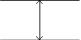ISWAP¶

In :
iswap()
q = QubitCircuit(2, reverse_states=False)
q.png
Out: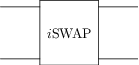SQRTiSWAP¶

In :
sqrtiswap()
Out:
Quantum object: dims = [[2, 2], [2, 2]], shape = (4, 4), type = oper, isherm = False\begin{equation*}\left(\begin{array}{*{11}c}1.0 & 0.0 & 0.0 & 0.0\\0.0 & 0.707 & 0.707j & 0.0\\0.0 & 0.707j & 0.707 & 0.0\\0.0 & 0.0 & 0.0 & 1.0\\\end{array}\right)\end{equation*}

SQRTSWAP¶

In :
sqrtswap()
Out:
Quantum object: dims = [[2, 2], [2, 2]], shape = (4, 4), type = oper, isherm = False\begin{equation*}\left(\begin{array}{*{11}c}1.0 & 0.0 & 0.0 & 0.0\\0.0 & (0.500+0.500j) & (0.500-0.500j) & 0.0\\0.0 & (0.500-0.500j) & (0.500+0.500j) & 0.0\\0.0 & 0.0 & 0.0 & 1.0\\\end{array}\right)\end{equation*}

SQRTNOT¶

In :
sqrtnot()
Out:
Quantum object: dims = [, ], shape = (2, 2), type = oper, isherm = False\begin{equation*}\left(\begin{array}{*{11}c}(0.500+0.500j) & (0.500-0.500j)\\(0.500-0.500j) & (0.500+0.500j)\\\end{array}\right)\end{equation*}

In :
snot()
Out:
Quantum object: dims = [, ], shape = (2, 2), type = oper, isherm = True\begin{equation*}\left(\begin{array}{*{11}c}0.707 & 0.707\\0.707 & -0.707\\\end{array}\right)\end{equation*}

PHASEGATE¶

In :
phasegate(pi/2)
Out:
Quantum object: dims = [, ], shape = (2, 2), type = oper, isherm = False\begin{equation*}\left(\begin{array}{*{11}c}1.0 & 0.0\\0.0 & 1.0j\\\end{array}\right)\end{equation*}

GLOBALPHASE¶

In :
globalphase(pi/2)
Out:
Quantum object: dims = [, ], shape = (2, 2), type = oper, isherm = False\begin{equation*}\left(\begin{array}{*{11}c}1.0j & 0.0\\0.0 & 1.0j\\\end{array}\right)\end{equation*}

Mølmer–Sørensen gate¶

In :
molmer_sorensen(pi/2)
Out:
Quantum object: dims = [[2, 2], [2, 2]], shape = (4, 4), type = oper, isherm = False\begin{equation*}\left(\begin{array}{*{11}c}0.707 & 0.0 & 0.0 & -0.707j\\0.0 & 0.707 & -0.707j & 0.0\\0.0 & -0.707j & 0.707 & 0.0\\-0.707j & 0.0 & 0.0 & 0.707\\\end{array}\right)\end{equation*}

Qubit rotation gate¶

In :
qrot(pi/2, pi/4)
Out:
Quantum object: dims = [, ], shape = (2, 2), type = oper, isherm = False\begin{equation*}\left(\begin{array}{*{11}c}0.707 & (-0.500-0.500j)\\(0.500-0.500j) & 0.707\\\end{array}\right)\end{equation*}

Expanding gates to larger qubit registers¶

The example above show how to generate matrice representations of the gates implemented in QuTiP, in their minimal qubit requirements. If the same gates is to be represented in a qubit register of size $N$, the optional keywork argument N can be specified when calling the gate function. For example, to generate the matrix for the CNOT gate for a $N=3$ bit register:

In :
cnot(N=3)
Out:
Quantum object: dims = [[2, 2, 2], [2, 2, 2]], shape = (8, 8), type = oper, isherm = True\begin{equation*}\left(\begin{array}{*{11}c}1.0 & 0.0 & 0.0 & 0.0 & 0.0 & 0.0 & 0.0 & 0.0\\0.0 & 1.0 & 0.0 & 0.0 & 0.0 & 0.0 & 0.0 & 0.0\\0.0 & 0.0 & 1.0 & 0.0 & 0.0 & 0.0 & 0.0 & 0.0\\0.0 & 0.0 & 0.0 & 1.0 & 0.0 & 0.0 & 0.0 & 0.0\\0.0 & 0.0 & 0.0 & 0.0 & 0.0 & 0.0 & 1.0 & 0.0\\0.0 & 0.0 & 0.0 & 0.0 & 0.0 & 0.0 & 0.0 & 1.0\\0.0 & 0.0 & 0.0 & 0.0 & 1.0 & 0.0 & 0.0 & 0.0\\0.0 & 0.0 & 0.0 & 0.0 & 0.0 & 1.0 & 0.0 & 0.0\\\end{array}\right)\end{equation*}
In :
q = QubitCircuit(3, reverse_states=False)
q.png
Out: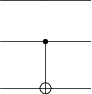Furthermore, the control and target qubits (when applicable) can also be similarly specified using keyword arguments control and target (or in some cases controls or targets):

In :
cnot(N=3, control=2, target=0)
Out:
Quantum object: dims = [[2, 2, 2], [2, 2, 2]], shape = (8, 8), type = oper, isherm = True\begin{equation*}\left(\begin{array}{*{11}c}1.0 & 0.0 & 0.0 & 0.0 & 0.0 & 0.0 & 0.0 & 0.0\\0.0 & 0.0 & 0.0 & 0.0 & 0.0 & 1.0 & 0.0 & 0.0\\0.0 & 0.0 & 1.0 & 0.0 & 0.0 & 0.0 & 0.0 & 0.0\\0.0 & 0.0 & 0.0 & 0.0 & 0.0 & 0.0 & 0.0 & 1.0\\0.0 & 0.0 & 0.0 & 0.0 & 1.0 & 0.0 & 0.0 & 0.0\\0.0 & 1.0 & 0.0 & 0.0 & 0.0 & 0.0 & 0.0 & 0.0\\0.0 & 0.0 & 0.0 & 0.0 & 0.0 & 0.0 & 1.0 & 0.0\\0.0 & 0.0 & 0.0 & 1.0 & 0.0 & 0.0 & 0.0 & 0.0\\\end{array}\right)\end{equation*}
In :
q = QubitCircuit(3, reverse_states=False)
q.png
Out: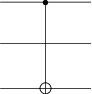Setup of a Qubit Circuit¶

The gates implemented in QuTiP can be used to build any qubit circuit using the class QubitCircuit. The output can be obtained in the form of a unitary matrix or a latex representation.

In the following example, we take a SWAP gate. It is known that a swap gate is equivalent to three CNOT gates applied in the given format.

In :
N = 2
qc0 = QubitCircuit(N)
qc0.png
Out: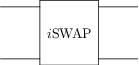In :
U_list0 = qc0.propagators()
U0 = gate_sequence_product(U_list0)
U0
Out:
Quantum object: dims = [[2, 2], [2, 2]], shape = (4, 4), type = oper, isherm = False\begin{equation*}\left(\begin{array}{*{11}c}1.0 & 0.0 & 0.0 & 0.0\\0.0 & 0.0 & 1.0j & 0.0\\0.0 & 1.0j & 0.0 & 0.0\\0.0 & 0.0 & 0.0 & 1.0\\\end{array}\right)\end{equation*}
In :
qc1 = QubitCircuit(N)
qc1.png
Out: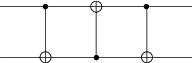In :
U_list1 = qc1.propagators()
U1 = gate_sequence_product(U_list1)
U1
Out:
Quantum object: dims = [[2, 2], [2, 2]], shape = (4, 4), type = oper, isherm = True\begin{equation*}\left(\begin{array}{*{11}c}1.0 & 0.0 & 0.0 & 0.0\\0.0 & 0.0 & 1.0 & 0.0\\0.0 & 1.0 & 0.0 & 0.0\\0.0 & 0.0 & 0.0 & 1.0\\\end{array}\right)\end{equation*}

In place of manually converting the SWAP gate to CNOTs, it can be automatically converted using an inbuilt function in QubitCircuit

In :
qc2 = qc0.resolve_gates("CNOT")
qc2.png
Out: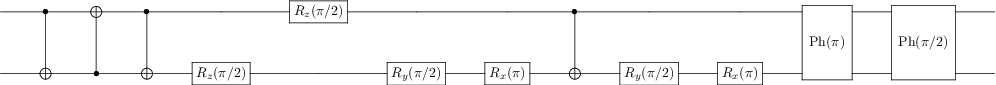In :
U_list2 = qc2.propagators()
U2 = gate_sequence_product(U_list2)
U2
Out:
Quantum object: dims = [[2, 2], [2, 2]], shape = (4, 4), type = oper, isherm = False\begin{equation*}\left(\begin{array}{*{11}c}1.0 & 0.0 & 0.0 & 0.0\\0.0 & 0.0 & 1.0j & 0.0\\0.0 & 1.0j & 0.0 & 0.0\\0.0 & 0.0 & 0.0 & 1.0\\\end{array}\right)\end{equation*}

From QuTiP 4.4, we can also add gate at arbitrary position in a circuit.

In :
qc1.png
Out: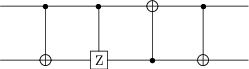Example of basis transformation¶

In :
qc3 = QubitCircuit(3)
qc3.png
Out: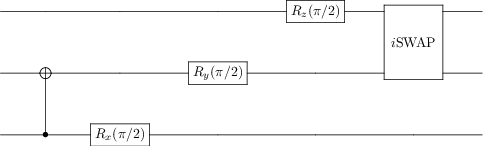In :
U3 = gate_sequence_product(qc3.propagators())
U3
Out:
Quantum object: dims = [[2, 2, 2], [2, 2, 2]], shape = (8, 8), type = oper, isherm = False\begin{equation*}\left(\begin{array}{*{11}c}(0.354-0.354j) & 0.0 & (-0.354+0.354j) & 0.0 & (0.354+0.354j) & 0.0 & (-0.354-0.354j) & 0.0\\(0.354+0.354j) & 0.0 & (0.354+0.354j) & 0.0 & (0.354-0.354j) & 0.0 & (0.354-0.354j) & 0.0\\0.0 & (-0.354+0.354j) & 0.0 & (0.354-0.354j) & 0.0 & (-0.354-0.354j) & 0.0 & (0.354+0.354j)\\0.0 & (0.354+0.354j) & 0.0 & (0.354+0.354j) & 0.0 & (0.354-0.354j) & 0.0 & (0.354-0.354j)\\(-0.354-0.354j) & 0.0 & (0.354+0.354j) & 0.0 & (-0.354+0.354j) & 0.0 & (0.354-0.354j) & 0.0\\(0.354-0.354j) & 0.0 & (0.354-0.354j) & 0.0 & (0.354+0.354j) & 0.0 & (0.354+0.354j) & 0.0\\0.0 & (0.354+0.354j) & 0.0 & (-0.354-0.354j) & 0.0 & (0.354-0.354j) & 0.0 & (-0.354+0.354j)\\0.0 & (0.354-0.354j) & 0.0 & (0.354-0.354j) & 0.0 & (0.354+0.354j) & 0.0 & (0.354+0.354j)\\\end{array}\right)\end{equation*}

The transformation can either be only in terms of 2-qubit gates:¶

In :
qc4 = qc3.resolve_gates("CNOT")
qc4.png
Out: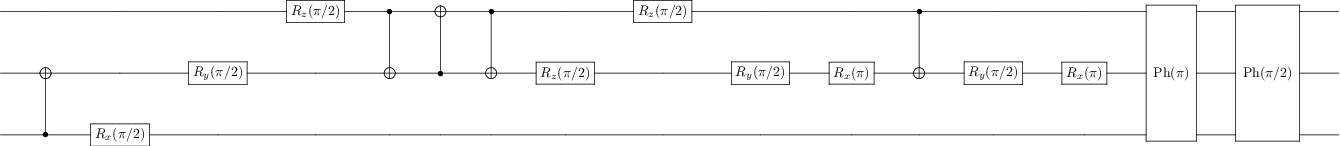In :
U4 = gate_sequence_product(qc4.propagators())
U4
Out:
Quantum object: dims = [[2, 2, 2], [2, 2, 2]], shape = (8, 8), type = oper, isherm = False\begin{equation*}\left(\begin{array}{*{11}c}(0.354-0.354j) & 0.0 & (-0.354+0.354j) & 0.0 & (0.354+0.354j) & 0.0 & (-0.354-0.354j) & 0.0\\(0.354+0.354j) & 0.0 & (0.354+0.354j) & 0.0 & (0.354-0.354j) & 0.0 & (0.354-0.354j) & 0.0\\0.0 & (-0.354+0.354j) & 0.0 & (0.354-0.354j) & 0.0 & (-0.354-0.354j) & 0.0 & (0.354+0.354j)\\0.0 & (0.354+0.354j) & 0.0 & (0.354+0.354j) & 0.0 & (0.354-0.354j) & 0.0 & (0.354-0.354j)\\(-0.354-0.354j) & 0.0 & (0.354+0.354j) & 0.0 & (-0.354+0.354j) & 0.0 & (0.354-0.354j) & 0.0\\(0.354-0.354j) & 0.0 & (0.354-0.354j) & 0.0 & (0.354+0.354j) & 0.0 & (0.354+0.354j) & 0.0\\0.0 & (0.354+0.354j) & 0.0 & (-0.354-0.354j) & 0.0 & (0.354-0.354j) & 0.0 & (-0.354+0.354j)\\0.0 & (0.354-0.354j) & 0.0 & (0.354-0.354j) & 0.0 & (0.354+0.354j) & 0.0 & (0.354+0.354j)\\\end{array}\right)\end{equation*}
In :
qc5 = qc3.resolve_gates("ISWAP")
qc5.png
Out: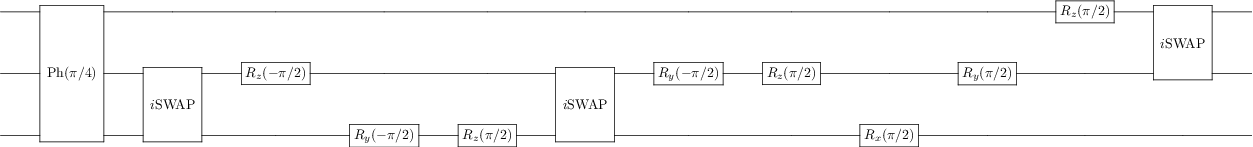In :
U5 = gate_sequence_product(qc5.propagators())
U5
Out:
Quantum object: dims = [[2, 2, 2], [2, 2, 2]], shape = (8, 8), type = oper, isherm = False\begin{equation*}\left(\begin{array}{*{11}c}(0.354-0.354j) & 0.0 & (-0.354+0.354j) & 0.0 & (0.354+0.354j) & 0.0 & (-0.354-0.354j) & 0.0\\(0.354+0.354j) & 0.0 & (0.354+0.354j) & 0.0 & (0.354-0.354j) & 0.0 & (0.354-0.354j) & 0.0\\0.0 & (-0.354+0.354j) & 0.0 & (0.354-0.354j) & 0.0 & (-0.354-0.354j) & 0.0 & (0.354+0.354j)\\0.0 & (0.354+0.354j) & 0.0 & (0.354+0.354j) & 0.0 & (0.354-0.354j) & 0.0 & (0.354-0.354j)\\(-0.354-0.354j) & 0.0 & (0.354+0.354j) & 0.0 & (-0.354+0.354j) & 0.0 & (0.354-0.354j) & 0.0\\(0.354-0.354j) & 0.0 & (0.354-0.354j) & 0.0 & (0.354+0.354j) & 0.0 & (0.354+0.354j) & 0.0\\0.0 & (0.354+0.354j) & 0.0 & (-0.354-0.354j) & 0.0 & (0.354-0.354j) & 0.0 & (-0.354+0.354j)\\0.0 & (0.354-0.354j) & 0.0 & (0.354-0.354j) & 0.0 & (0.354+0.354j) & 0.0 & (0.354+0.354j)\\\end{array}\right)\end{equation*}

Or the transformation can be in terms of any 2 single qubit rotation gates along with the 2-qubit gate.¶

In :
qc6 = qc3.resolve_gates(["ISWAP", "RX", "RY"])
qc6.png
Out: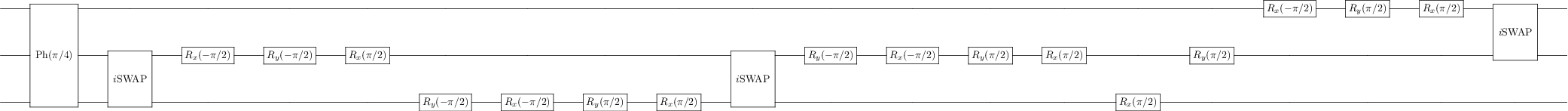In :
U6 = gate_sequence_product(qc6.propagators())
U6
Out:
Quantum object: dims = [[2, 2, 2], [2, 2, 2]], shape = (8, 8), type = oper, isherm = False\begin{equation*}\left(\begin{array}{*{11}c}(0.354-0.354j) & 0.0 & (-0.354+0.354j) & 0.0 & (0.354+0.354j) & 0.0 & (-0.354-0.354j) & 0.0\\(0.354+0.354j) & 0.0 & (0.354+0.354j) & 0.0 & (0.354-0.354j) & 0.0 & (0.354-0.354j) & 0.0\\0.0 & (-0.354+0.354j) & 0.0 & (0.354-0.354j) & 0.0 & (-0.354-0.354j) & 0.0 & (0.354+0.354j)\\0.0 & (0.354+0.354j) & 0.0 & (0.354+0.354j) & 0.0 & (0.354-0.354j) & 0.0 & (0.354-0.354j)\\(-0.354-0.354j) & 0.0 & (0.354+0.354j) & 0.0 & (-0.354+0.354j) & 0.0 & (0.354-0.354j) & 0.0\\(0.354-0.354j) & 0.0 & (0.354-0.354j) & 0.0 & (0.354+0.354j) & 0.0 & (0.354+0.354j) & 0.0\\0.0 & (0.354+0.354j) & 0.0 & (-0.354-0.354j) & 0.0 & (0.354-0.354j) & 0.0 & (-0.354+0.354j)\\0.0 & (0.354-0.354j) & 0.0 & (0.354-0.354j) & 0.0 & (0.354+0.354j) & 0.0 & (0.354+0.354j)\\\end{array}\right)\end{equation*}
In :
qc7 = qc3.resolve_gates(["CNOT", "RZ", "RX"])
qc7.png
Out: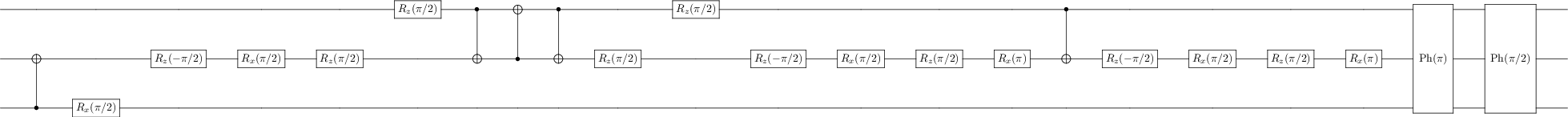In :
U7 = gate_sequence_product(qc7.propagators())
U7
Out:
Quantum object: dims = [[2, 2, 2], [2, 2, 2]], shape = (8, 8), type = oper, isherm = False\begin{equation*}\left(\begin{array}{*{11}c}(0.354-0.354j) & 0.0 & (-0.354+0.354j) & 0.0 & (0.354+0.354j) & 0.0 & (-0.354-0.354j) & 0.0\\(0.354+0.354j) & 0.0 & (0.354+0.354j) & 0.0 & (0.354-0.354j) & 0.0 & (0.354-0.354j) & 0.0\\0.0 & (-0.354+0.354j) & 0.0 & (0.354-0.354j) & 0.0 & (-0.354-0.354j) & 0.0 & (0.354+0.354j)\\0.0 & (0.354+0.354j) & 0.0 & (0.354+0.354j) & 0.0 & (0.354-0.354j) & 0.0 & (0.354-0.354j)\\(-0.354-0.354j) & 0.0 & (0.354+0.354j) & 0.0 & (-0.354+0.354j) & 0.0 & (0.354-0.354j) & 0.0\\(0.354-0.354j) & 0.0 & (0.354-0.354j) & 0.0 & (0.354+0.354j) & 0.0 & (0.354+0.354j) & 0.0\\0.0 & (0.354+0.354j) & 0.0 & (-0.354-0.354j) & 0.0 & (0.354-0.354j) & 0.0 & (-0.354+0.354j)\\0.0 & (0.354-0.354j) & 0.0 & (0.354-0.354j) & 0.0 & (0.354+0.354j) & 0.0 & (0.354+0.354j)\\\end{array}\right)\end{equation*}

Interactions between non-adjacent qubits can be resolved by QubitCircuit to a series of adjacent interactions, which is useful for systems such as spin chain models.

In :
qc8 = QubitCircuit(3)
qc8.png
Out: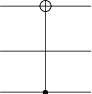In :
U8 = gate_sequence_product(qc8.propagators())
U8
Out:
Quantum object: dims = [[2, 2, 2], [2, 2, 2]], shape = (8, 8), type = oper, isherm = True\begin{equation*}\left(\begin{array}{*{11}c}1.0 & 0.0 & 0.0 & 0.0 & 0.0 & 0.0 & 0.0 & 0.0\\0.0 & 1.0 & 0.0 & 0.0 & 0.0 & 0.0 & 0.0 & 0.0\\0.0 & 0.0 & 1.0 & 0.0 & 0.0 & 0.0 & 0.0 & 0.0\\0.0 & 0.0 & 0.0 & 1.0 & 0.0 & 0.0 & 0.0 & 0.0\\0.0 & 0.0 & 0.0 & 0.0 & 0.0 & 1.0 & 0.0 & 0.0\\0.0 & 0.0 & 0.0 & 0.0 & 1.0 & 0.0 & 0.0 & 0.0\\0.0 & 0.0 & 0.0 & 0.0 & 0.0 & 0.0 & 0.0 & 1.0\\0.0 & 0.0 & 0.0 & 0.0 & 0.0 & 0.0 & 1.0 & 0.0\\\end{array}\right)\end{equation*}
In :
qc9.gates
Out:
[Gate(SWAP, targets=[0, 1], controls=None, classical controls=None, control_value=None),
Gate(CNOT, targets=, controls=, classical controls=None, control_value=None),
Gate(SWAP, targets=[0, 1], controls=None, classical controls=None, control_value=None)]
In :
U9 = gate_sequence_product(qc9.propagators())
U9
Out:
Quantum object: dims = [[2, 2, 2], [2, 2, 2]], shape = (8, 8), type = oper, isherm = True\begin{equation*}\left(\begin{array}{*{11}c}1.0 & 0.0 & 0.0 & 0.0 & 0.0 & 0.0 & 0.0 & 0.0\\0.0 & 1.0 & 0.0 & 0.0 & 0.0 & 0.0 & 0.0 & 0.0\\0.0 & 0.0 & 1.0 & 0.0 & 0.0 & 0.0 & 0.0 & 0.0\\0.0 & 0.0 & 0.0 & 1.0 & 0.0 & 0.0 & 0.0 & 0.0\\0.0 & 0.0 & 0.0 & 0.0 & 0.0 & 1.0 & 0.0 & 0.0\\0.0 & 0.0 & 0.0 & 0.0 & 1.0 & 0.0 & 0.0 & 0.0\\0.0 & 0.0 & 0.0 & 0.0 & 0.0 & 0.0 & 0.0 & 1.0\\0.0 & 0.0 & 0.0 & 0.0 & 0.0 & 0.0 & 1.0 & 0.0\\\end{array}\right)\end{equation*}
In :
qc10 = qc9.resolve_gates("CNOT")
qc10.png
Out: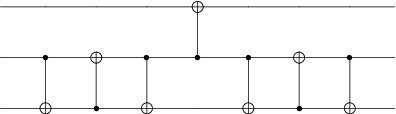In :
U10 = gate_sequence_product(qc10.propagators())
U10
Out:
Quantum object: dims = [[2, 2, 2], [2, 2, 2]], shape = (8, 8), type = oper, isherm = True\begin{equation*}\left(\begin{array}{*{11}c}1.0 & 0.0 & 0.0 & 0.0 & 0.0 & 0.0 & 0.0 & 0.0\\0.0 & 1.0 & 0.0 & 0.0 & 0.0 & 0.0 & 0.0 & 0.0\\0.0 & 0.0 & 1.0 & 0.0 & 0.0 & 0.0 & 0.0 & 0.0\\0.0 & 0.0 & 0.0 & 1.0 & 0.0 & 0.0 & 0.0 & 0.0\\0.0 & 0.0 & 0.0 & 0.0 & 0.0 & 1.0 & 0.0 & 0.0\\0.0 & 0.0 & 0.0 & 0.0 & 1.0 & 0.0 & 0.0 & 0.0\\0.0 & 0.0 & 0.0 & 0.0 & 0.0 & 0.0 & 0.0 & 1.0\\0.0 & 0.0 & 0.0 & 0.0 & 0.0 & 0.0 & 1.0 & 0.0\\\end{array}\right)\end{equation*}

Adding gate in the middle of a circuit¶

From QuTiP 4.4 one can add a gate at an arbitrary position of a circuit. All one needs to do is to specify the parameter index. With this, we can also add the same gate at multiple positions at the same time.

In :
qc = QubitCircuit(1)
qc.gates
Out:
[Gate(RY, targets=, controls=None, classical controls=None, control_value=None),
Gate(RX, targets=, controls=None, classical controls=None, control_value=None),
Gate(RX, targets=, controls=None, classical controls=None, control_value=None)]

User defined gates¶

From QuTiP 4.4 on, user defined gates can be defined by a python function that takes at most one parameter and return a Qobj, the dimension of the Qobj has to match the qubit system.

In :
def user_gate1(arg_value):
# controlled rotation X
mat = np.zeros((4, 4), dtype=np.complex)
mat[0, 0] = mat[1, 1] = 1.
mat[2:4, 2:4] = rx(arg_value)
return Qobj(mat, dims=[[2, 2], [2, 2]])

def user_gate2():
# S gate
mat = np.array([[1.,   0],
[0., 1.j]])
return Qobj(mat, dims=[, ])

To let the QubitCircuit process those gates, we need to modify its attribute QubitCircuit.user_gates, which is a python dictionary in the form {name: gate_function}.

In :
qc = QubitCircuit(2)
qc.user_gates = {"CTRLRX": user_gate1,
"S"     : user_gate2}

When calling the add_gate method, the target qubits and the argument need to be given.

In :
# qubit 0 controls qubit 1
# qubit 1 controls qubit 0
# a gate can also be added using the Gate class
g_T = Gate("S", targets=)
props = qc.propagators()
<ipython-input-60-0c4e722f5dae>:3: DeprecationWarning: np.complex is a deprecated alias for the builtin complex. To silence this warning, use complex by itself. Doing this will not modify any behavior and is safe. If you specifically wanted the numpy scalar type, use np.complex128 here.
Deprecated in NumPy 1.20; for more details and guidance: https://numpy.org/devdocs/release/1.20.0-notes.html#deprecations
mat = np.zeros((4, 4), dtype=np.complex)
In :
props # qubit 0 controls qubit 1
Out:
Quantum object: dims = [[2, 2], [2, 2]], shape = (4, 4), type = oper, isherm = False\begin{equation*}\left(\begin{array}{*{11}c}1.0 & 0.0 & 0.0 & 0.0\\0.0 & 1.0 & 0.0 & 0.0\\0.0 & 0.0 & 0.707 & -0.707j\\0.0 & 0.0 & -0.707j & 0.707\\\end{array}\right)\end{equation*}
In :
props # qubit 1 controls qubit 0
Out:
Quantum object: dims = [[2, 2], [2, 2]], shape = (4, 4), type = oper, isherm = False\begin{equation*}\left(\begin{array}{*{11}c}1.0 & 0.0 & 0.0 & 0.0\\0.0 & 0.707 & 0.0 & -0.707j\\0.0 & 0.0 & 1.0 & 0.0\\0.0 & -0.707j & 0.0 & 0.707\\\end{array}\right)\end{equation*}
In :
props # S  gate acts on qubit 1
Out:
Quantum object: dims = [[2, 2], [2, 2]], shape = (4, 4), type = oper, isherm = False\begin{equation*}\left(\begin{array}{*{11}c}1.0 & 0.0 & 0.0 & 0.0\\0.0 & 1.0j & 0.0 & 0.0\\0.0 & 0.0 & 1.0 & 0.0\\0.0 & 0.0 & 0.0 & 1.0j\\\end{array}\right)\end{equation*}

Software versions¶

In :
from qutip.ipynbtools import version_table
version_table()
Out:
SoftwareVersion
QuTiP4.6.0+c003ff5
Numpy1.20.1
SciPy1.5.3
matplotlib3.3.0
Cython0.29.21
Number of CPUs12
BLAS InfoGeneric
IPython7.16.1
Python3.8.6 | packaged by conda-forge | (default, Oct 7 2020, 18:22:52) [MSC v.1916 64 bit (AMD64)]
OSnt [win32]
Mon Apr 12 19:47:05 2021 W. Europe Daylight Time